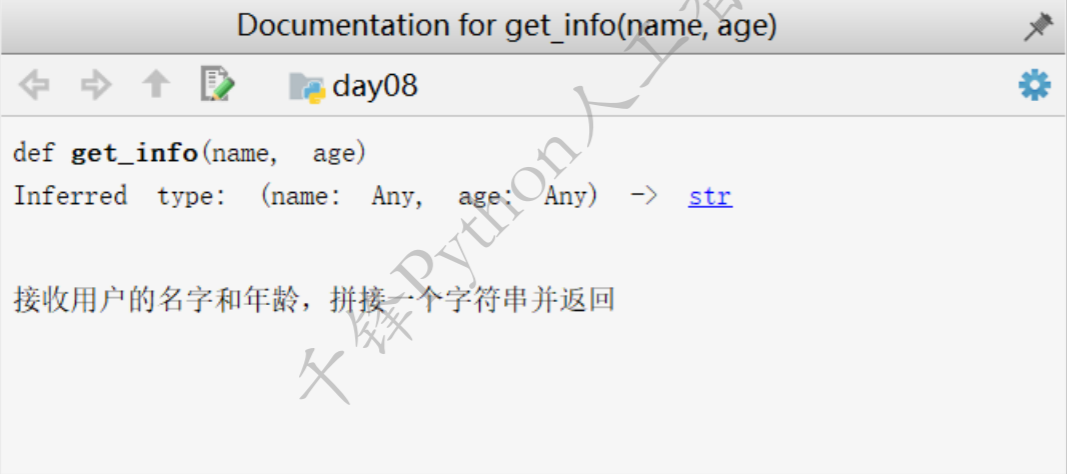# 递归列出目录里的文件、匿名函数

+关注继续查看

#!/usr/bin/python

import os

import sys

def print_file(path):

lidir=os.listdir(path)

dirs=[i for i in lidir if os.path.isdir(os.path.join(path,i))]

files=[i for i in lidir if os.path.isfile(os.path.join(path,i))]

if files:

for i in files:

print os.path.join(path,i)

if dirs:

for j in dirs:

print_file(os.path.join(path,j))

print_file(sys.argv)

...:     return x + y

...:

Out: 7

In : r=lambda x,y:x+y # lambda 语句中，冒号前是参数，可以有多个，用逗号隔开，冒号右边是返回值，lambda 语句构建的其实是一个函数对象

In : r(4,5)

Out: 9567 0Linux系统下统计目录及其子目录文件个数
1037 01004 02014秋C++ 第12周项目 C++函数新特征与递归函数

1130 07.4 匿名函数和高阶函数
1、匿名函数：没有名字的函数 def sum(s, y):     return x * y m = lambda x, y: x * y print(m) print(m(4, 5)) 2、sorted() 高阶函数 对字典进行排序 mm=dict(a=1,c=10,b=4,d=9) for i in mm:     print(i) for j in mm.
659 0《HTML与CSS入门经典（第8版）》——2.3 理解Web服务器上放置文件的位置
1270 0MySQL配置文件my.ini参数注释说明
2269 0﻿﻿ dd if=***.img bs=1 skip=172 > ***_nohead.bin
405 0lambda匿名函数使用
#!/usr/bin/pythonfun=lambda x:x*x-xprint fun(3)
438 0
14527

0

《SaaS模式云原生数据仓库应用场景实践》

《看见新力量：二》电子书